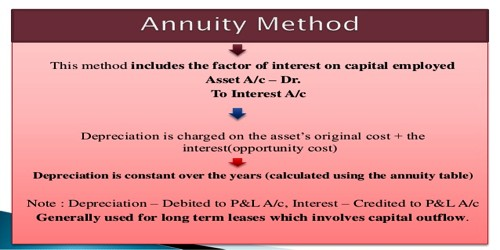# Annuity Method for Calculating Depreciation

Annuity Method for Calculating Depreciation

The annuity method considers that the business besides loosing the original cost of the asset in terms of depreciation and also looses interest on the amount used for buying the asset. This is based on the assumption that the amount invested in the asset would have earned in case the same amount would have been invested in some other form of investment. The annual amount of depreciation is determined with the help of annuity table. This method is used to calculate depreciation amount on lease.

Annuity method is not suitable for assets like plant and machinery because it requires frequent additions and extensions. It is particularly applicable to those assets whose cost is heavy and life is long and fixed, e.g. leasehold property, land and building etc.

Merits (Annuity Method):

• The amount of depreciation to be charged is ascertained from Annuity Tables. Therefore, this method is scientific.
• This method provides for recovery of invested capital along with interest. This is a great advantage.
• This method is most proper to such assets which require heavy primary investment.

Demerits:

• Calculation of depreciation becomes very complicated when additions are made to assets.
• Calculation of interest is arbitrary.
• This system is not at all suitable for those assets which are of small value.